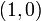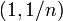# Closed infinite broom

The closed infinite broom is the subset of the Euclidean plane obtained as the union of the following line segments: the line segment joining$(0,0)$ to$(1,0)$, and the line segment joining$(0,0)$ to$(1,1/n)$ where$n$ varies over the natural numbers.